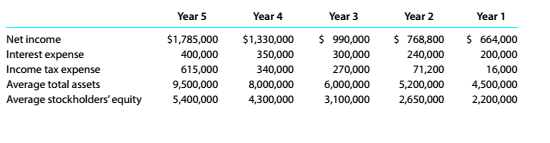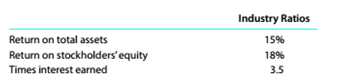Chapter 9, Problem 9.5.1P

Chapter
Section
Textbook Problem

Trend analysis Critelli Company has provided the following comparative information:You have been asked to evaluate the historical performance of the company over the last five years.Selected industry ratios have remained relatively steady at the following levels for the last five years:Instructions I.Prepare three line graphs, with the ratio on the vertical axis and the years on die horizontal axis for the following three ratios (rounded to one decimal place): A.Return on total assets b.Return on stockholders' equity c.Times interest earned Display both the company ratio and the industry- benchmark on each graph. That is. each graph should have two lines.

To determine

(a)

Concept Introduction:

Return on total assets is a ratio used for profitability analysis. It shows the ability of the firm to earn profit from its assets.

It can be determined as follows:

Return on Total Assets = Net Income + Interest ExpenseAverage Total Assets

To represent:

The Company's return on total assets ratio as well as industry benchmark on a line graph.

Explanation
 Year Net Income(A) Interest Expense (B) Average Total Assets (C) Return on Total Assets (A+B)/C Year 5 $1,785,000$400,000 $9,500,000 0.23 Year 4$1,330,000 $350,000$8,000,000 0...
To determine

(b)

Introduction:

Return on shareholders' equityis a ratio used for profitability analysis. It shows the ability of the firm to earn profit from investment by the shareholders.

It can be determined as follows:

Return on shareholders' Equity = Net IncomeAverage Total Stockholders' Equity

To prepare:

The Company's return on shareholders' equity ratio as well as industry benchmark on line graph.

To determine

(c)

Introduction:

Time interest earned:

It is used to measure a company's ability to meet interest obligations on its debt. It can be calculated using following formula

Time interest earned=Earning before interest and taxes  Total Interest payable

To prepare:

The Company's time interest earned ratio as well as industry benchmark on line graph.

Still sussing out bartleby?

Check out a sample textbook solution.

See a sample solution

The Solution to Your Study Problems

Bartleby provides explanations to thousands of textbook problems written by our experts, many with advanced degrees!

Get Started

CORPORATE VALUATION Smith Technologies is expected to generate 150 million in free cash flow next year, and FCF...

Fundamentals of Financial Management, Concise Edition (with Thomson ONE - Business School Edition, 1 term (6 months) Printed Access Card) (MindTap Course List)

Should an economic model describe reality exactly?

Principles of Microeconomics (MindTap Course List)

Why is productivity important?

Essentials of Economics (MindTap Course List)

For a stock to be in equilibrium, what two conditions must hold?

Fundamentals of Financial Management (MindTap Course List)